# Let f(x) = x + 12x + 37. On which of the following intervals is the...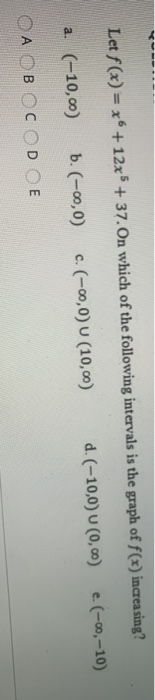Let f(x) = x + 12x + 37. On which of the following intervals is the graph of f(x) increasing? d. (-10,0) 0 (0, 0) е. (-ю, -10) а (-10, ою) ь. (-0,0) с. (-00,0) U (10,0) ОА ОВ OcOD OE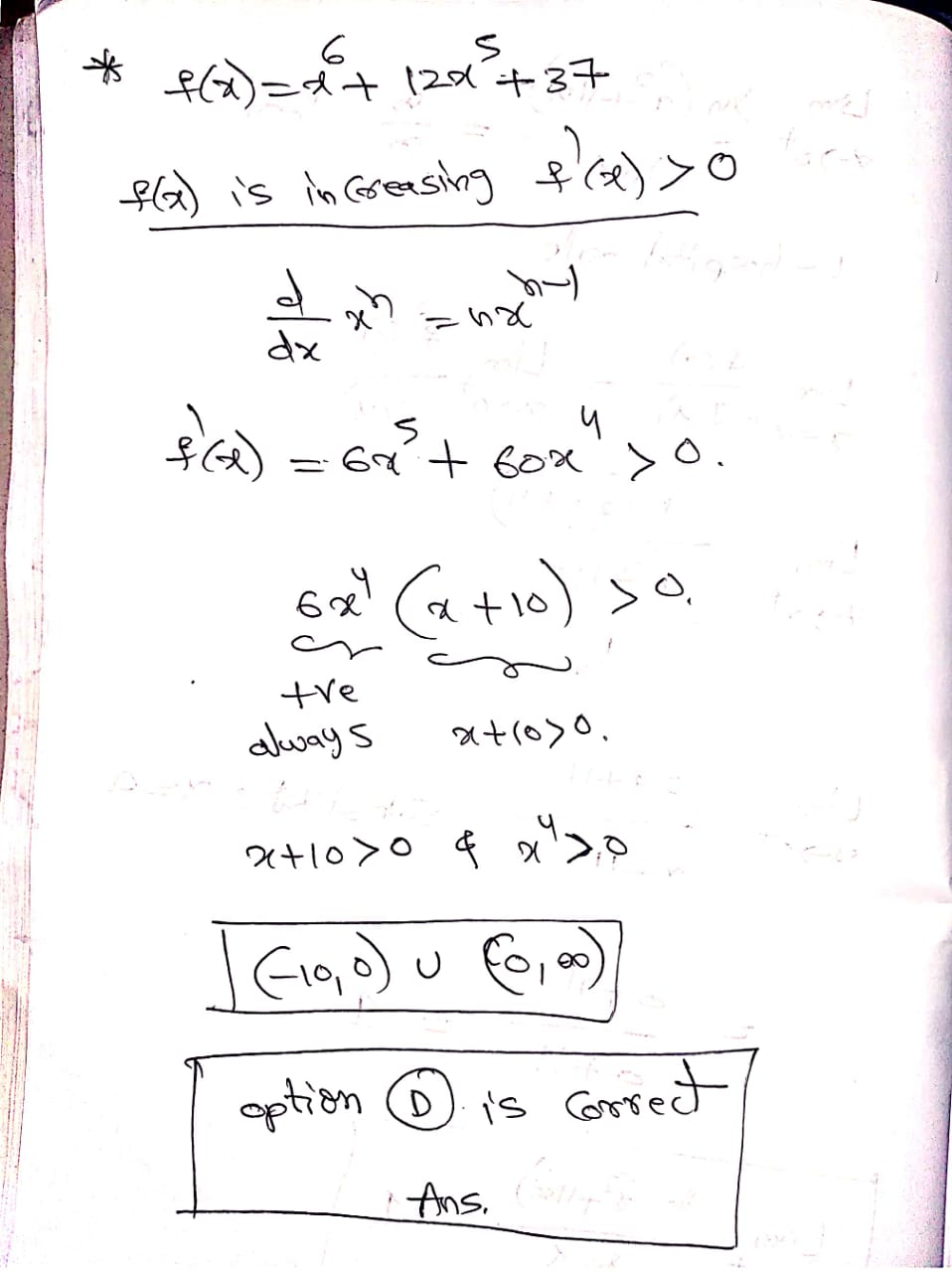#### Earn Coin

Coins can be redeemed for fabulous gifts.

Similar Homework Help Questions
• ### Find the intervals on which f(x) is increasing and the intervals on which f(x) is decreasing....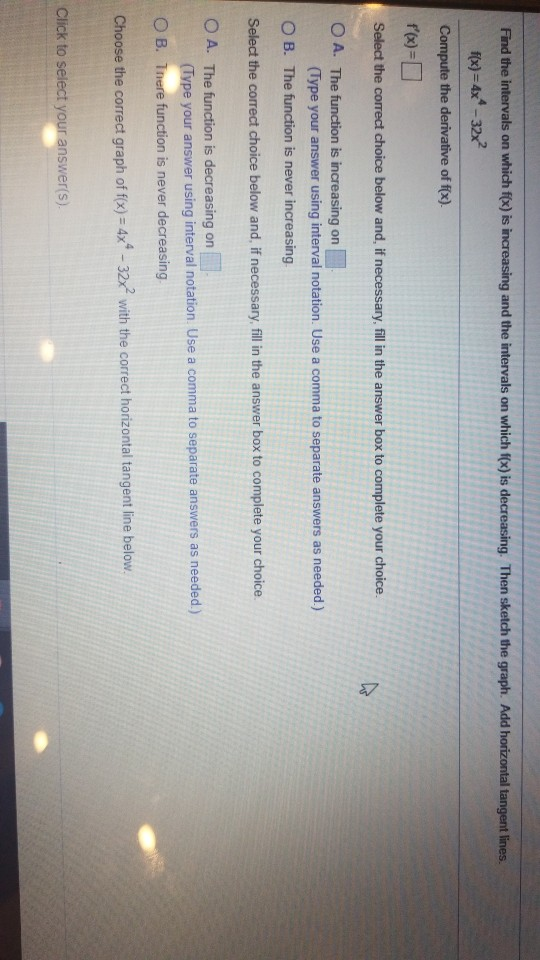Find the intervals on which f(x) is increasing and the intervals on which f(x) is decreasing. Then sketch the graph. Add horizontal tangent lines. f(x)=4x4 -32x2 Compute the derivative of f(x). f'(x)= Select the correct choice below and, if necessary, fill in the answer box to complete your choice. O A. The function is increasing on (Type your answer using interval notation. Use a comma to separate answers as needed.) OB. The function is never increasing Select the correct choice...

• ### show work 2. Let f(x) = x* – 18x²+4. a) Find the intervals on which f...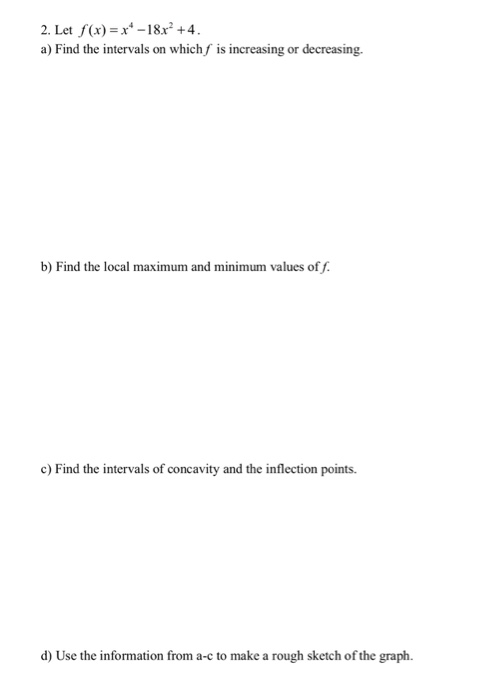show work 2. Let f(x) = x* – 18x²+4. a) Find the intervals on which f is increasing or decreasing. b) Find the local maximum and minimum values off. c) Find the intervals of concavity and the inflection points. d) Use the information from a-c to make a rough sketch of the graph.

• ### show work 2. Let f(x)=x* - 18x+4. a) Find the intervals on which f is increasing...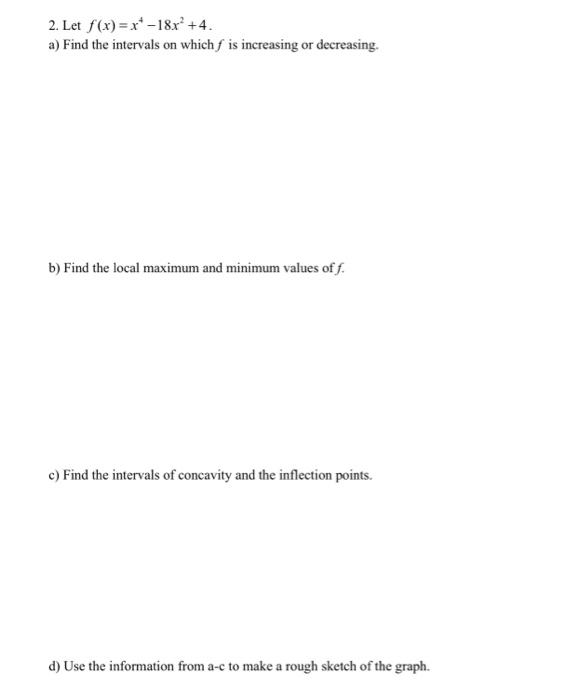show work 2. Let f(x)=x* - 18x+4. a) Find the intervals on which f is increasing or decreasing. b) Find the local maximum and minimum values of f. c) Find the intervals of concavity and the inflection points. d) Use the information from a-c to make a rough sketch of the graph.

• ### (15 points) Let f(t) = 21/3(x +4). (a) Find the intervals on which f is increasing...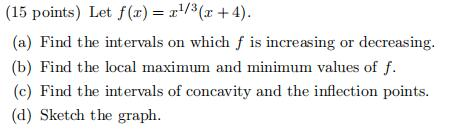(15 points) Let f(t) = 21/3(x +4). (a) Find the intervals on which f is increasing or decreasing, (b) Find the local maximum and minimum values of f. (c) Find the intervals of concavity and the inflection points. (d) Sketch the graph.

• ### 12. Determine the intervals on which the function f(x) = 3x5 - 10x3 + 12x -...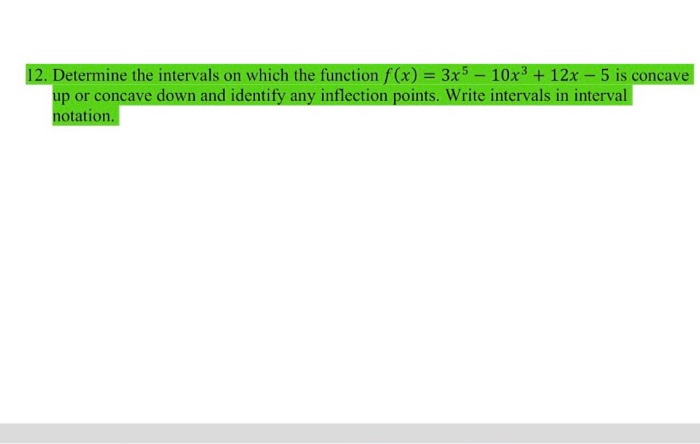12. Determine the intervals on which the function f(x) = 3x5 - 10x3 + 12x - 5 is concave up or concave down and identify any inflection points. Write intervals in interval notation.

• ### 8. Let ー2 ift_0 f(t)if 0 <t 3-t if t > 1 Sketch by hand a graph of y - f(t). Let g(x) f(t)dt be defined for -2 4. Using FTC find g'(x). Then determine the sign of g(x) and find intervals o...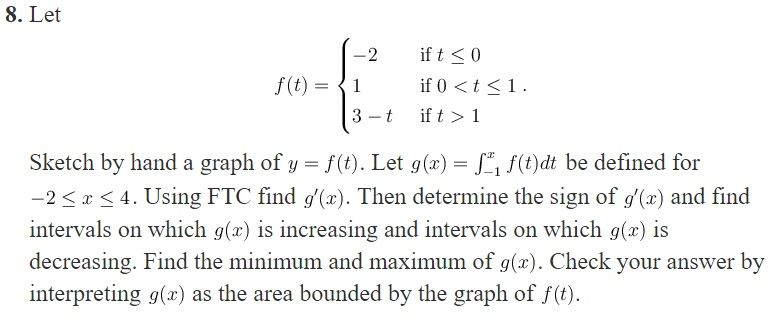8. Let ー2 ift_0 f(t)if 0 <t 3-t if t > 1 Sketch by hand a graph of y - f(t). Let g(x) f(t)dt be defined for -2 4. Using FTC find g'(x). Then determine the sign of g(x) and find intervals on which 9(2) is increasing and intervals on which g(x) is decreasing. Find the minimum and maximum of g(x). Check your answer by interpreting g(x) as the area bounded by the graph of f(t). 8. Let ー2 ift_0...

• ### Q-5: [5x1 marks] Let f(x) = 10 + (x – 2)4 a) Find f'(x) and f'(x)....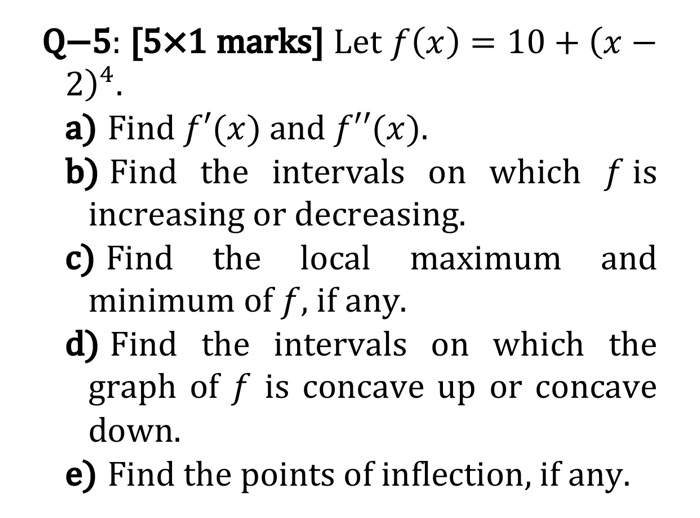Q-5: [5x1 marks] Let f(x) = 10 + (x – 2)4 a) Find f'(x) and f'(x). b) Find the intervals on which f is increasing or decreasing. c) Find the local maximum and minimum of f, if any. d) Find the intervals on which the graph of f is concave up or concave down. e) Find the points of inflection, if any.

• ### The graph of y = f(x) is shown to the right. Identify the intervals on which...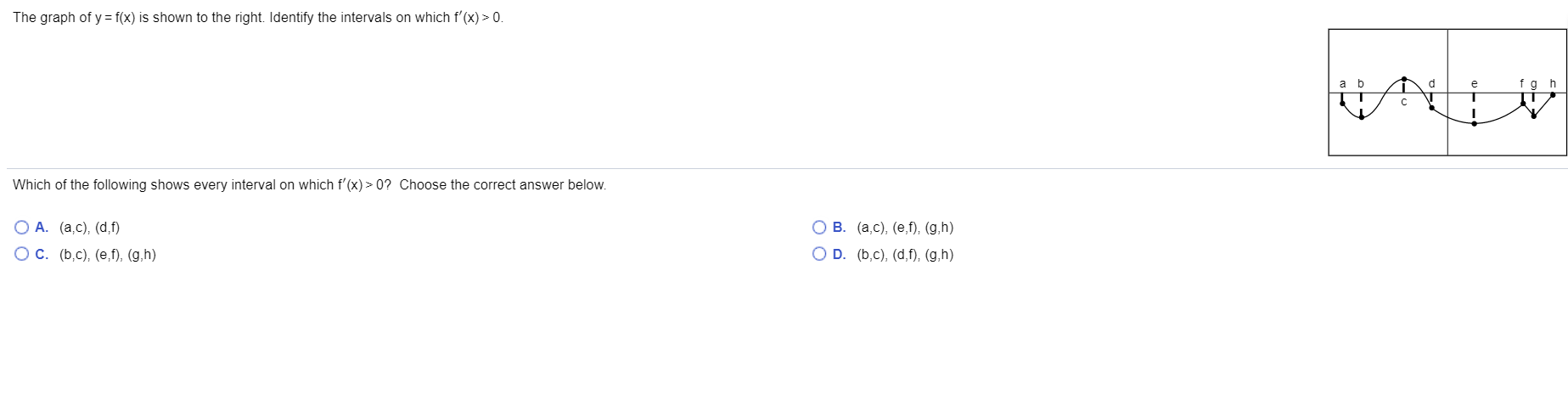The graph of y = f(x) is shown to the right. Identify the intervals on which f'(x) > 0. a b d fah IT с Which of the following shows every interval on which f'(x) > 0? Choose the correct answer below. O A. (ac), (d.f) O C. (5.c), (e,f), (g,h) O B. (a.c), (e,f), (g,h) OD. (5.c), (d,f), (g,h)

• ### The graph of y=f(x) is shown to the right. Identify the intervals on which f(x) is...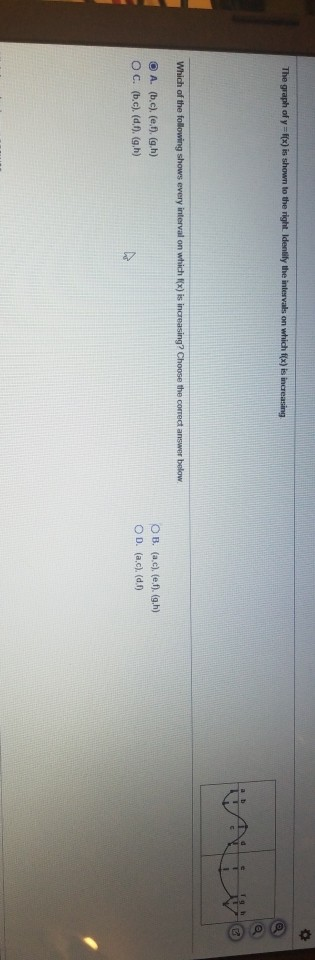The graph of y=f(x) is shown to the right. Identify the intervals on which f(x) is increasing 1h Which of the following shows every interval on which f(x) is increasing? Choose the correct answer below A. (b.c), (e,t). (g.h) O C. (5.c), (0,1),(g,h) OB. (a.c), (e.f). (g.h) OD. (a.c), (d.1)

• ### Let F(x) = f f (t) dt for 2 in the interval (0,3), where f (t)is...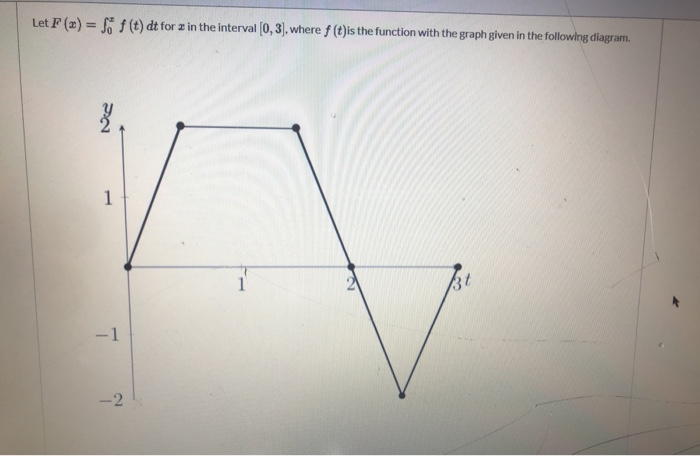Let F(x) = f f (t) dt for 2 in the interval (0,3), where f (t)is the function with the graph given in the following diagram. Ne 1 37 - 1 -2 Which of the following statements are true? Select all that apply. Fhas a local maximum at 2. F has a local minimum at 2. F is increasing on the intervals (0,0.5) and (2.5, 3). Fis decreasing on the interval (1.5, 2.5).

Free Homework App